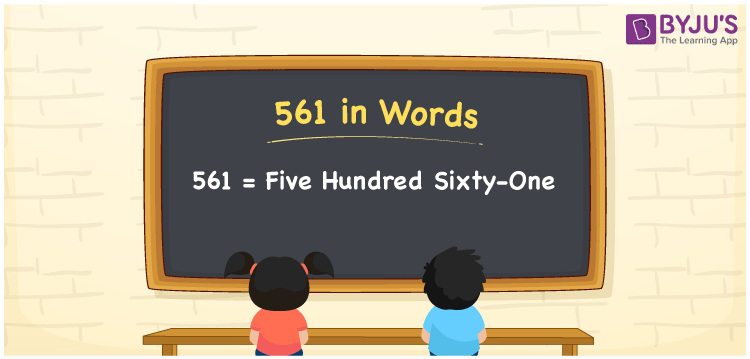# 561 in Words

The number 561 in words is “five hundred sixty-one”. In Mathematics, the number 561 is a natural number and it appears after the number 560, but just before 562. Also, the numeral 561 is a cardinal number. We can easily write the number 561 in words using the place value system.

 561 in Words: Five Hundred Sixty-one. Five Hundred Sixty-one in Numerical Form: 561.

## 561 in English Words## How to Write 561 in Words?

The place value table for the numeral 561 is provided below.

 Hundreds Tens Ones 5 6 1

The expanded form of 561 is as follows:

= 5 × Hundred + 6 × Ten + 1 × One

= 5 × 100 + 6 × 10 + 1 × 1

= 500 + 60 + 1

= 561

= Five hundred sixty-one

Hence, 561 in words is five hundred sixty-one.

561 in words – Five hundred sixty-one

Is 561 an odd number? – Yes

Is 561 an even number? – No

Is 561 a perfect square number? – No

Is 561 a perfect cube number? – No

Is 561 a prime number? – No

Is 561 a composite number? – Yes

## Frequently Asked Questions on 561 in Words

### Write 561 in English words.

561 in words is five hundred sixty-one.

### Simplify 500 + 61, and express it in words.

Simplifying 500 + 61, we get 561. Hence, 561 in words is five hundred sixty-one.

### Is 561 a composite number?

Yes, 561 is a composite number.